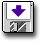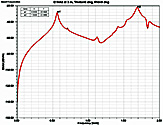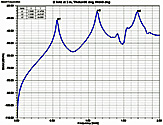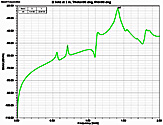Modeling a Powerbus with HFSS (version 11.1.3)

 Geometry and setup Double-sided PCB: Size: 125 mm × 100 mm × 1 mm Top and bottom metal: PEC Dielectric: FR4, εr = 4.5, dielectric loss tangent = 0.015 Solution type: Driven terminalExcitation: Voltage source (1 V, 50 ohms) Boundary: RadiationAnalysis Setup: Solution Frequency: 1.15 GHz Maximum Number of Passes: 50 Maximum ΔS: 0.01 Do Lambda Refinement: 0.4 Maximum Refinement Passes: 20% Sweep: Sweep type: Discrete Frequency Setup: 5 MHz - 2 GHz, Step Size = 5 MHzhfss_powerbus.zip Simulation result Simulation Time: 44 mins 50 secsNumber of passes completed: 7Number of tetrahedra: 3776 Decisions the user must make that affect the accuracy of the result Solution type: driven terminal Location of absorbing boundary: cylinder, radius=150 mm, height=300 mm Maximum ΔS: default = 0.02, this model = 0.01 Do lambda refinement: default = 0.333, this model =0.4 Maximum refinement passes: default=30%, this model=20% Comments How did we select the solution type? Two kinds of solution types are available in HFSS, Driven Modal and Driven Terminal. We used the Driven Modal solution type to calculate the input impedance and the Driven Terminal solution type to calculate the far-zone radiation. How did we select the excitation type? In HFSS, impedance matrix parameters are computed from the S-parameters and port impedances. Since lumped ports compute S-parameters directly at the port, it is more efficient to use a lumped port when you want HFSS to calculate the input impedance and assign a voltage source when you want to specify the voltage and direction of the electric field on a surface. In this model, the power bus is driven by a 50-ohm source. To simulate the source, an ideal voltage source was assigned to a rectangle from the edge of the upper layer to the RLC boundary. The RCL boundary was modeled as a 50-ohm resistor in series with the ideal voltage source.
 Screen shotsFig. 4. Electric field at 3 m, θ=0°, φ=0°Fig. 5. Electric field at 3 m, θ=90°, φ=0°Fig. 6. Electric field at 3 m, θ=90°, φ=90°